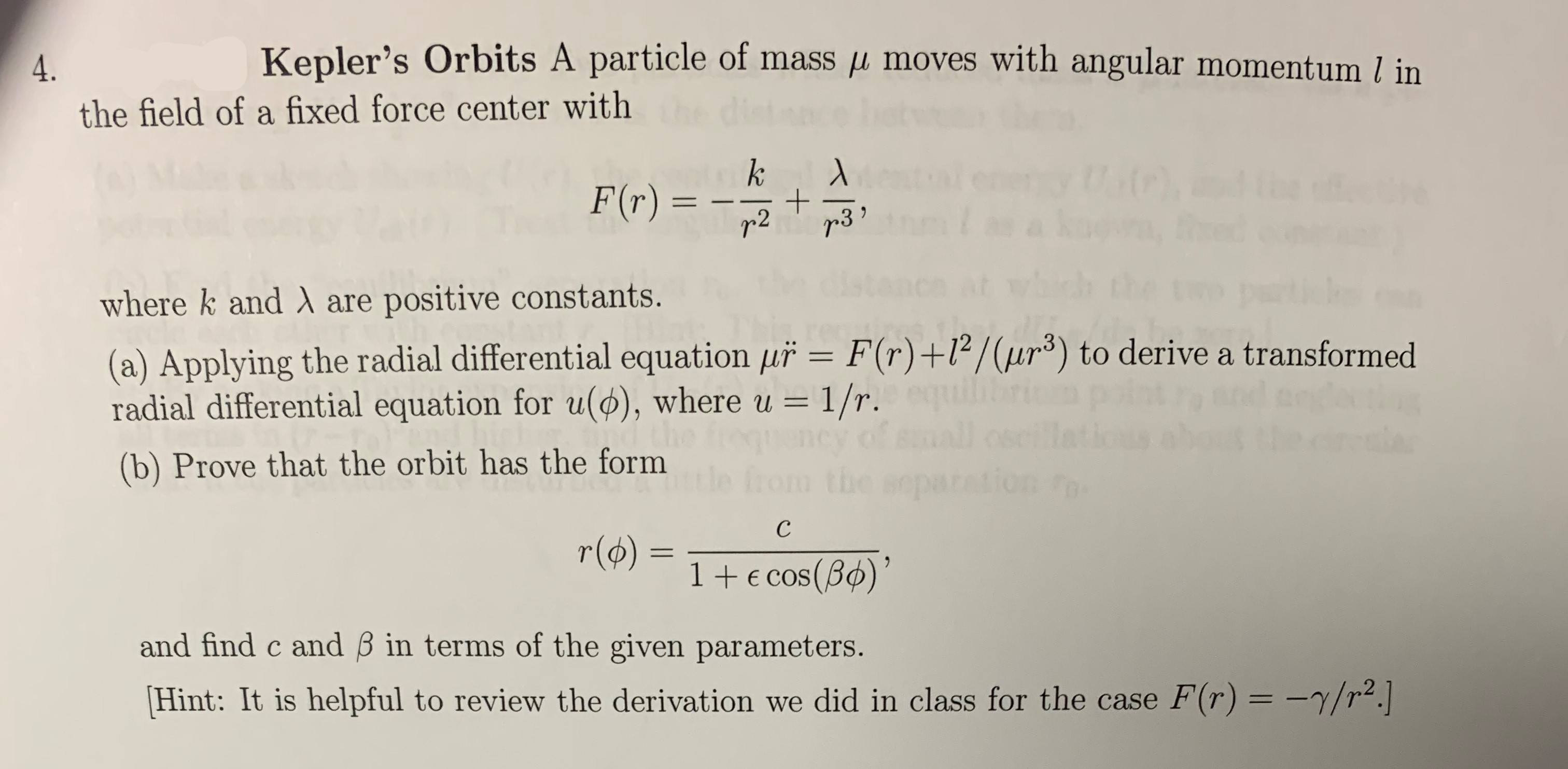# Kepler's Orbits A particle of mass p moves with angular momentum l inthe field of a fixed force center withkF(r) =+r3r 2where k and A are positive constants.(a) Applying the radial differential equation uř = F(r) +l2/(ur3) to derive a transformedradial differential equation for u(), where u = 1/r.(b) Prove that the orbit has the formtle from the separationсr(o)1+E cos (B)and find c and B in terms of the given parameters.Hint: It is helpful to review the derivation we did in class for the case F(r) = -7/r2.

Question
104 viewshelp_outlineImage TranscriptioncloseKepler's Orbits A particle of mass p moves with angular momentum l in the field of a fixed force center with k F(r) = + r3 r 2 where k and A are positive constants. (a) Applying the radial differential equation uř = F(r) +l2/(ur3) to derive a transformed radial differential equation for u(), where u = 1/r. (b) Prove that the orbit has the form tle from the separation с r(o) 1+E cos (B) and find c and B in terms of the given parameters. Hint: It is helpful to review the derivation we did in class for the case F(r) = -7/r2. fullscreen
check_circle

Step 1

(a) Using the given radial differential equation, the force in terms of u is,

Step 2

Transforming the differentials from r to u,

Step 3

Moreover...

### Want to see the full answer?

See Solution

#### Want to see this answer and more?

Solutions are written by subject experts who are available 24/7. Questions are typically answered within 1 hour.*

See Solution
*Response times may vary by subject and question.
Tagged in